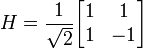# Quantum logic gates

You are probably aware of classical logic gates, such as the Boolean AND and OR gates. The input(s) and output(s) of a logic gate take values in the set {0, 1}, usually identified with ‘false’ and ‘true’, respectively.

One example with multiple inputs and outputs is the full adder, so called because of its ability to perform binary addition, which has three inputs (A, B, C) and two outputs (S, C’). It can be represented by a truth table, which gives the values of S and C’ given any combination of A, B, C:Thinking of this as a function from {0, 1}^3 to {0, 1}^2, we can also represent this as a binary matrix with eight columns and four rows, where every column contains a single ‘1’. This matrix is particularly interesting when it is a permutation matrix, corresponding to a bijective function. (Of course, this is only possible when the logic gate has equally many inputs and outputs.) These logic gates are known as reversible logic gates. It is a remarkable fact that reversible gates can perform any classical computation, and that there is a three-input universal gate, namely the Fredkin gate. This has three inputs, A, B and C, and three outputs, A’, B’, C’, with the following definition:

(A’, B’, C’) = (A, B, C) if C = 0, and (A’, B’, C’) = (B, A, C) if C = 1.

Anyway, if we generalise the definition of a logic gate to include any unitary matrix, rather than merely permutation matrices, then we obtain logic gates capable of creating a quantum superposition of outputs. For instance, the Hadamard gate has one input and one output, and corresponds to the following matrix:Another example is the controlled phase gate, which is a diagonal matrix with entries$(1, 1, 1, e^{i \theta})$. Conditional on the first qubit (quantum bit) being in state |1›, this rotates the Bloch sphere (space of possible states, which is isomorphic to the complex projective line or Riemann sphere) of the second qubit. It was used by Peter Shor to construct a circuit of logic gates capable of applying the quantum Fourier transform.

### Quantum computing

The primary interest in quantum logic gates derives from the fact that they can actually be implemented as physical systems, relying on quantum-mechanical phenomena such as quantum entanglement and the ability for the system to occupy a superposition of states. Certain problems have known solutions in probabilistic polynomial time with quantum computing, but no known probabilistic polynomial time solutions with classical computing. These include the problems of integer factorisation, discrete logarithm and its counterpart over elliptic curves. All of these are solved in cubic time by Shor’s algorithm.

At the moment, quantum computing is still very much in its infancy. Shor’s algorithm has indeed been implemented, but the largest number to be factored in this way was 21 (until recently, the record was 15). As technology improves, quantum computers executing Shor’s algorithm could considerably compromise RSA cryptography and elliptic curve cryptography. Indeed, I’m not sure whether there is a known public-key cryptosystem that isn’t known to have a polynomial-time quantum attack.

This entry was posted in Uncategorized. Bookmark the permalink.

### 0 Responses to Quantum logic gates

1.Perry Hooker says:
•apgoucher says:

Thanks!

2.wojowu says:

I know you have probably already been asked this question n! times, but I noticed there is 2 week delay with new cipher posts. Have you finished season II, or just again wait for some special date?

•apgoucher says:

I’ve finished Season II.